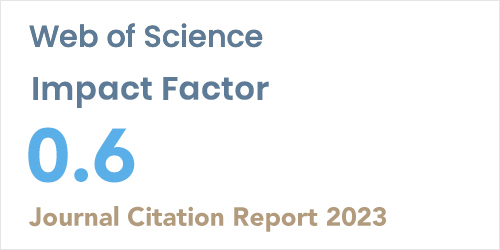ISSN: 1304-7191 | E-ISSN: 1304-7205
Volume: 41 Issue: 4 Year: 2023Global stability of Susceptible Diabetes Complication (SDC) model in discrete time
1Gumushane University, Mathematical Engineering Department, Gumushane, Turkey
Sigma J Eng Nat Sci 2021; 39(3): 290-312 DOI: 10.14744/sigma.2021.00018

#### Abstract

In this study, the mathematical model (DC) of diabetes disease is discussed. This model divides people into (D) uncomplicated and (C) complex diabetics two. In addition, diabetes is a disease known to be caused by genetic and environmental factors, and this factor is one of the main causes of genetic disorder at birth. Considering these two factors, the diabetes complication (SDC) model, which is sensitive from the diabetes complication (DC) model, is being developed. In this model, the responsive diabetes complication (SDC) model of a nonlinear system of differential equations is transformed into a discrete-time system of equations. The positivity and limitation of Model solutions were examined R0 the basic increment number is calculated. If R0 < 1, it has a global asymptotically stable balance for the situation where there is no genetic disorder at birth, and for R0 < 1, the system has an unstable balance. In addition, random behavior of the discrete model was examined for different probability distributions.